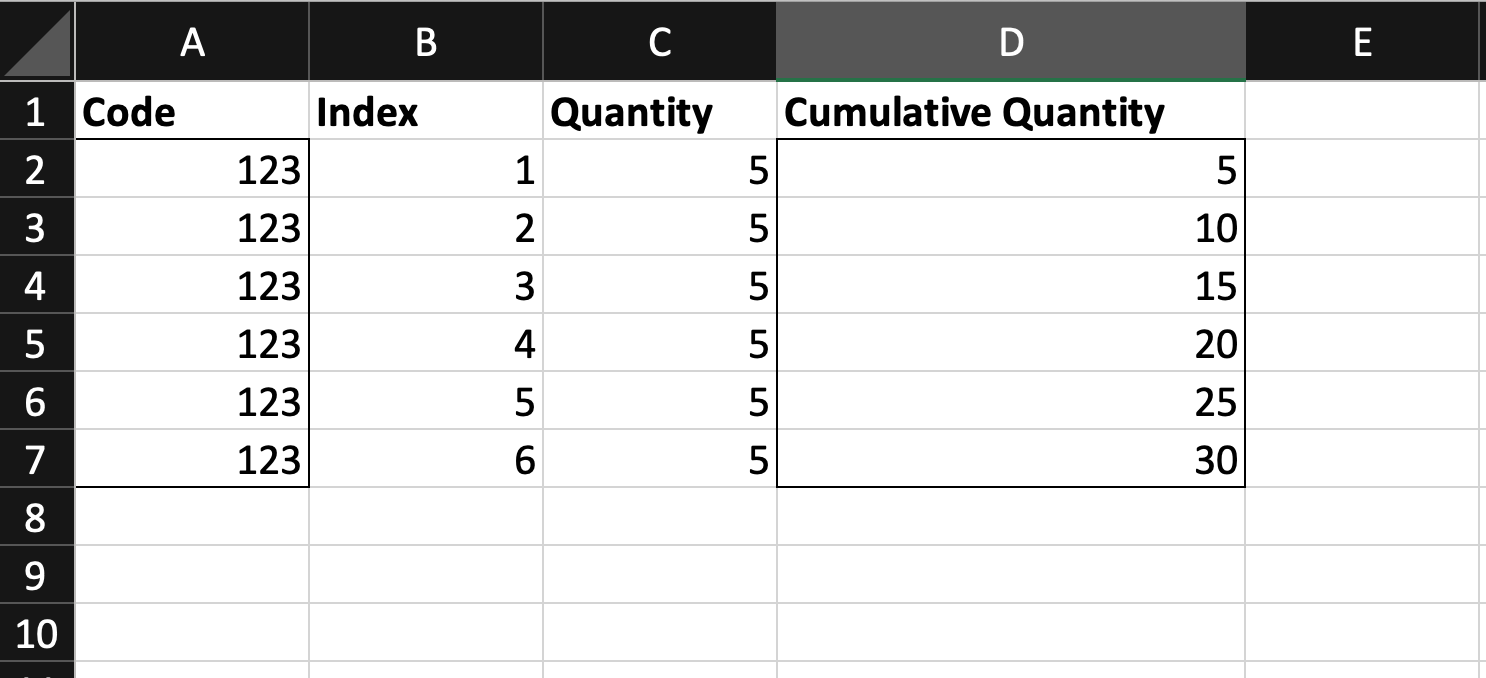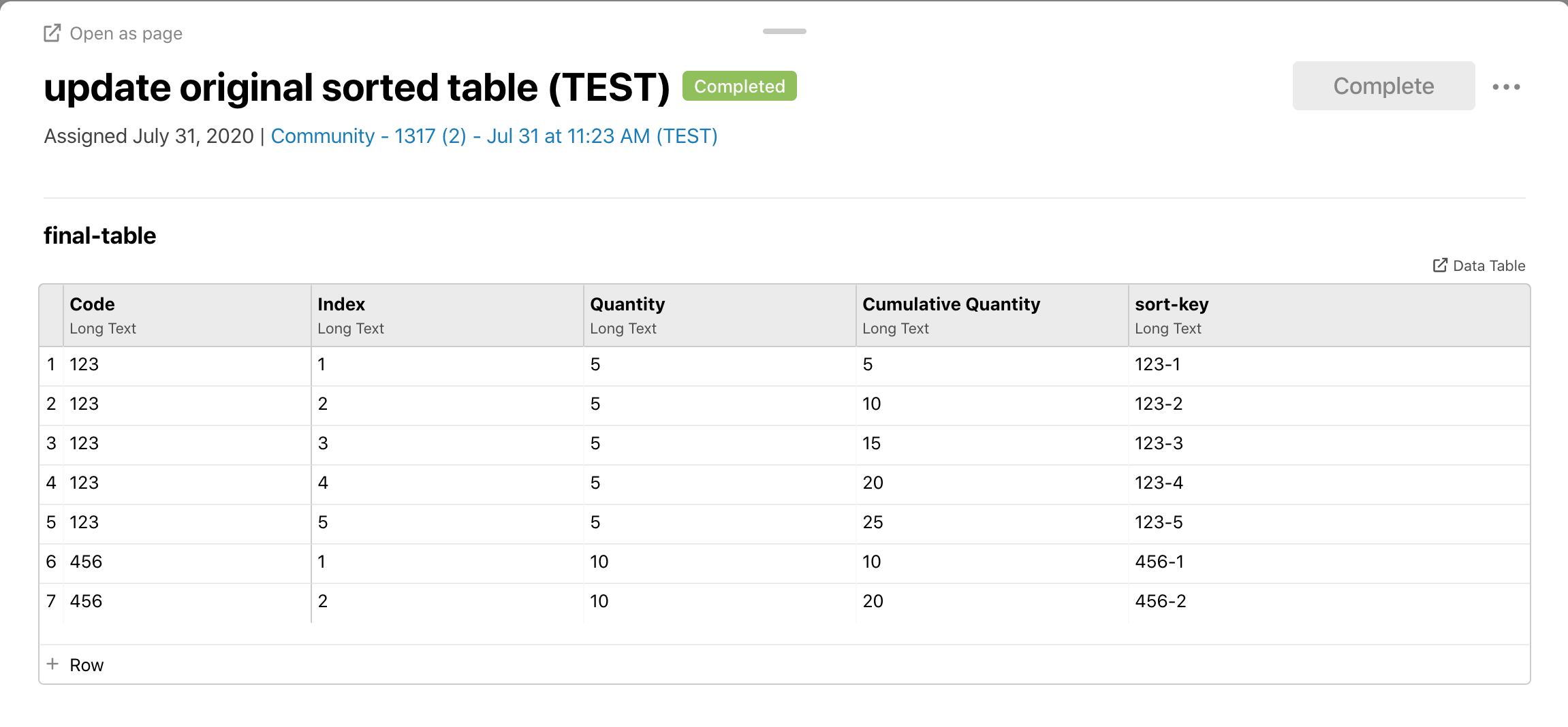# Table Formula - Cumulative Quantity by Partition

Can `SQL PARTITION BY` translate easily into JavaScript for use in Tables: Apply formulas to columns?

Example `SQL PARTITION BY`:

`SUM(Quantity) OVER (PARTITION BY Code Column ORDER BY Index Column) AS CUMULATIVE QUANTITY`

Based on the example SQL above and dataset image below, here is what we would like the JavaScript table formula to do:

For each matching Code Column row value, output a running total of the quantity associated to the code. Sort the result by the Index column.Tagged:

• @Jozef_783863 Perhaps this may be feasible if the formula is in an Excel template file, with Excel: Insert a data table into an Excel template? If that approach does not work, I wonder if it may somehow be possible with CSV: Summarize rows with formulas, but I'm not sure what the JavaScript would be.

• @Matt_727739, The 7 steps listed below output the following data table (workflow export attached):